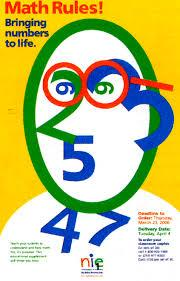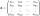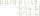# The inverse

The inverse matrix for matrix A has a determinant value of 0.333. What value has a determinant of the matrix A?

Correct result:

d =  3.003

#### Solution:

$d=1\mathrm{/}0.333=\frac{1000}{333}=3.003$We would be pleased if you find an error in the word problem, spelling mistakes, or inaccuracies and send it to us. Thank you!## Next similar math problems:

• Inverse matrixFind how many times is the larger determinant is the matrix A, which equals 9 as the determinant of its inverse matrix.
• Inverse matrixFind out inverse by Gauss elimination or by reduction method. A=[2/3. 1 -3. 1/3]
• The determinantThe determinant of the unit matrix equals 7. Check how many rows the A matrix contains.